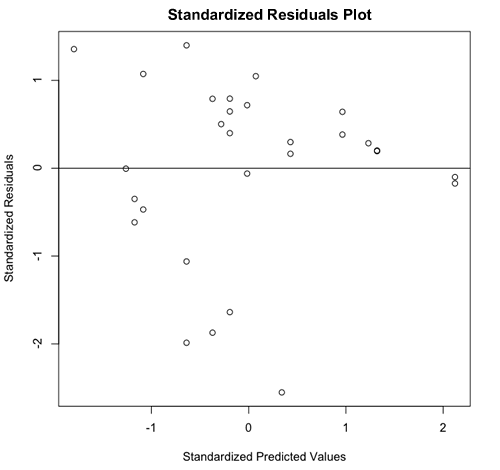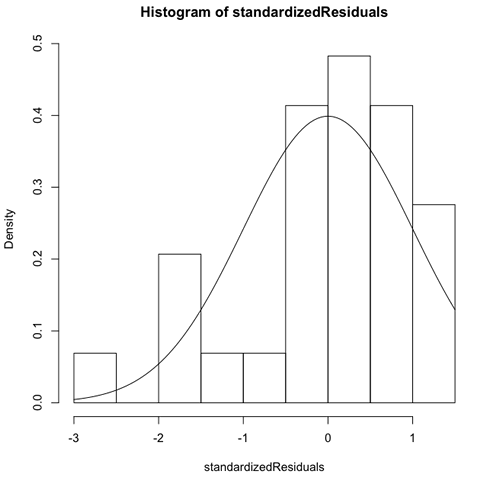[This article was first published on R Tutorial Series, and kindly contributed to R-bloggers]. (You can report issue about the content on this page here)
Want to share your content on R-bloggers? click here if you have a blog, or here if you don't.

An important aspect of regression involves assessing the tenability of the assumptions upon which its analyses are based. This tutorial will explore how R can help one scrutinize the regression assumptions of a model via its residuals plot, normality histogram, and PP plot.

### Tutorial Files

Before we begin, you may want to download the sample data (.csv) used in this tutorial. Be sure to right-click and save the file to your R working directory. This dataset contains information used to estimate undergraduate enrollment at the University of New Mexico (Office of Institutional Research, 1990). Note that all code samples in this tutorial assume that this data has already been read into an R variable and has been attached.

### Pre-Analysis Steps

Before testing the tenability of regression assumptions, we need to have a model. In the segment on simple linear regression, we created a single predictor model to estimate the fall undergraduate enrollment at the University of New Mexico. The complete code used to derive this model is provided in its respective tutorial. This article assumes that you are familiar with this models and how it was created. Therefore, a shorthand method for generating the model is displayed below.

1. > #create a linear model using lm(FORMULA, DATAVAR)
2. > #predict the fall enrollment (ROLL) using the unemployment rate (UNEM)
3. > linearModelVar <- lm(ROLL ~ UNEM, datavar)

### Tenability of Assumptions

#### Residuals Plot

A residuals plot can be used to assess the assumption that the variables have a linear relationship. The plot is formed by graphing the standardized residuals on the y-axis and the standardized predicted values on the x-axis. An optional horizontal line can be added to aid in interpreting the output.

The unstandardized predicted values can be generated using the predict(MODEL) function and the unstandardized residuals can be obtained via the resid(MODEL) function. In both cases, MODEL refers to the variable containing the regression model. Respectively, these values can be standardized by subtracting the mean and dividing by the standard deviation. The standardized data can be plotted using the plot() function (see Scatterplots). Lastly, abline(0,0) can be used to add a horizontal line to the plot. The code necessary to create a standardized residuals plot is presented below.

1. > #get unstandardized predicted and residual values
2. > unstandardizedPredicted <- predict(linearModelVar)
3. > unstandardizedResiduals <- resid(linearModelVar)
4. > #get standardized values
5. > standardizedPredicted <- (unstandardizedPredicted - mean(unstandardizedPredicted)) / sd(unstandardizedPredicted)
6. > standardizedResiduals <- (unstandardizedResiduals - mean(unstandardizedResiduals)) / sd(unstandardizedResiduals)
7. > #create standardized residuals plot
8. > plot(standardizedPredicted, standardizedResiduals, main = “Standardized Residuals Plot”, xlab = “Standardized Predicted Values”, ylab = “Standardized Residuals”)
9. > #add horizontal line
10. > abline(0,0)

Note that abline(0,0) must be executed after the plot is generated and while the Quartz window is open. The plot resulting from the preceding code is pictured below.In general, values that are close to the horizontal line are predicted well. The points above the line are underpredicted and the ones below the line are overpredicted. The linearity assumption is supported to the extent that the amount of points scattered above and below the line is equal.The residuals plot can also be used to test the homogeneity of variance (homoscedasticity ) assumption. Look at the vertical scatter at a given point along the x-axis. Now look at the vertical scatter across all points along the x-axis. The homogeneity of variance assumption is supported to the extent that the vertical scatter is the same across all x values.#### Residuals Histogram

A histogram can be used to assess the assumption that the residuals are normally distributed. In R, the hist(VAR, FREQ) function will produce the necessary graph, where VAR is the variable to be charted and FREQ is a boolean value indicating how frequencies are to be represented (true for counts, false for probabilities). Then, in similar fashion to abline(), a normal curve can be added to the histogram via the curve(EXPR, ADD) function, where EXPR is the type of curve to plot (here, “dnorm”) and ADD is a boolean value indicating whether or not to add the curve to the existing window. The following code demonstrates how to create a residuals histogram for our model.

1. > #create residuals histogram
2. > hist(standardizedResiduals, freq = FALSE)
3. > #add normal curve
4. > curve(dnorm, add = TRUE)

Note that curve() must be executed after the plot is generated and while the Quartz window is open. The plot resulting from the preceding code is pictured below.To the extent that the histogram matches the normal distribution, the residuals are normally distributed. This gives us an indication of how well our sample can predict a normal distribution in the population.#### PP Plot

A PP Plot can also be used to assess the assumption that the residuals are normally distributed. To create a PP Plot in R, we must first get the probability distribution using the pnorm(VAR) function, where VAR is the variable containing the residuals. Then we can use the plot(VAR, SORT) function to create the graph, where VAR is the variable containing the residuals and SORT makes use of our calculated probability distribution. Note that the ppoints() and length() functions are incorporated into the VAR parameter in this case. Lastly, the abline(0,1) function is used to draw a diagonal line across the plot for comparison purposes.

1. > #get probability distribution for residuals
2. > probDist <- pnorm(standardizedResiduals)
3. > #create PP plot
4. > plot(ppoints(length(standardizedResiduals)), sort(probDist), main = “PP Plot”, xlab = “Observed Probability”, ylab = “Expected Probability”)
5. > #add diagonal line
6. > abline(0,1)

Recall that abline(0,1) must be executed after the plot is generated and while the Quartz window is open. The plot resulting from the preceding code is pictured below.Here, the distribution is considered to be normal to the extent that the plotted points match the diagonal line.Complete Regression Assumptions Example

To see a complete example of how the regression assumptions of linearity, homoscedasticity, and normality can be analyzed visually in R, please download the regression assumptions example (.txt) file.

### References

Office of Institutional Research (1990). Enrollment Forecast [Data File]. Retrieved November 22, 2009 from http://lib.stat.cmu.edu/DASL/Datafiles/enrolldat.html

Svetina, D., & Levy, R. (2009). Regression Assumptions [Text File]. Retrieved December 7, 2009 from EDP 552: Multiple Regression and Correlation Methods [Protected Website].